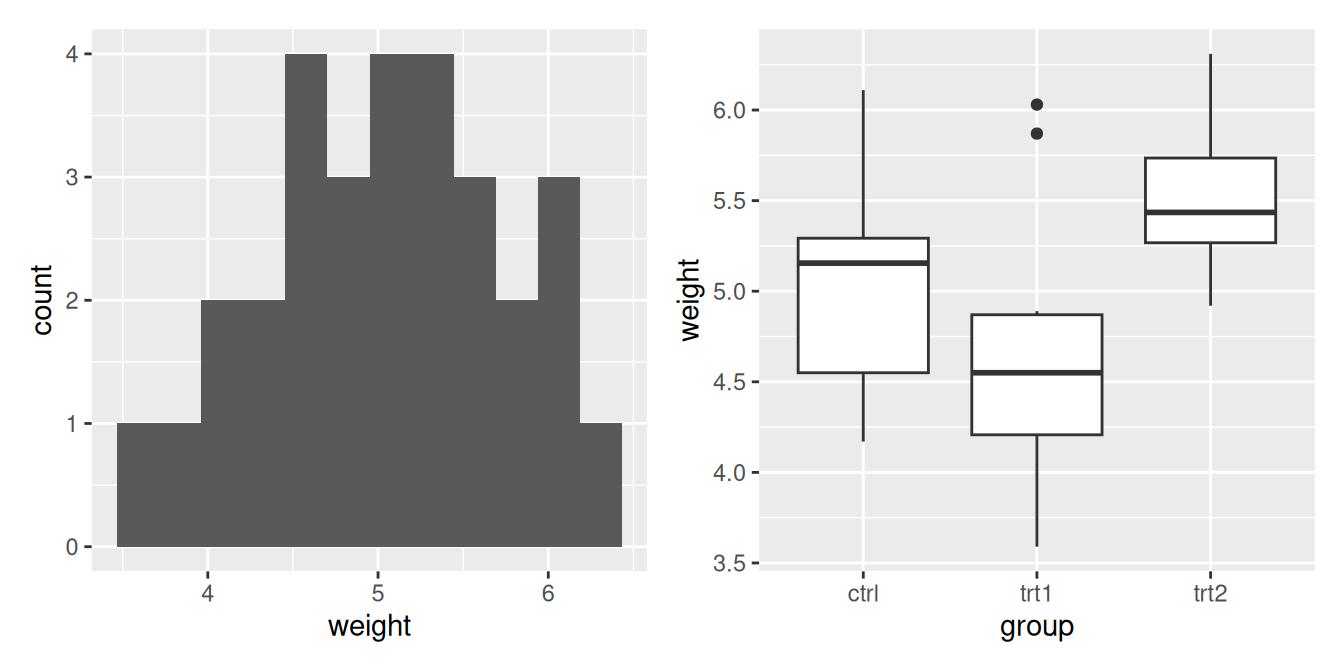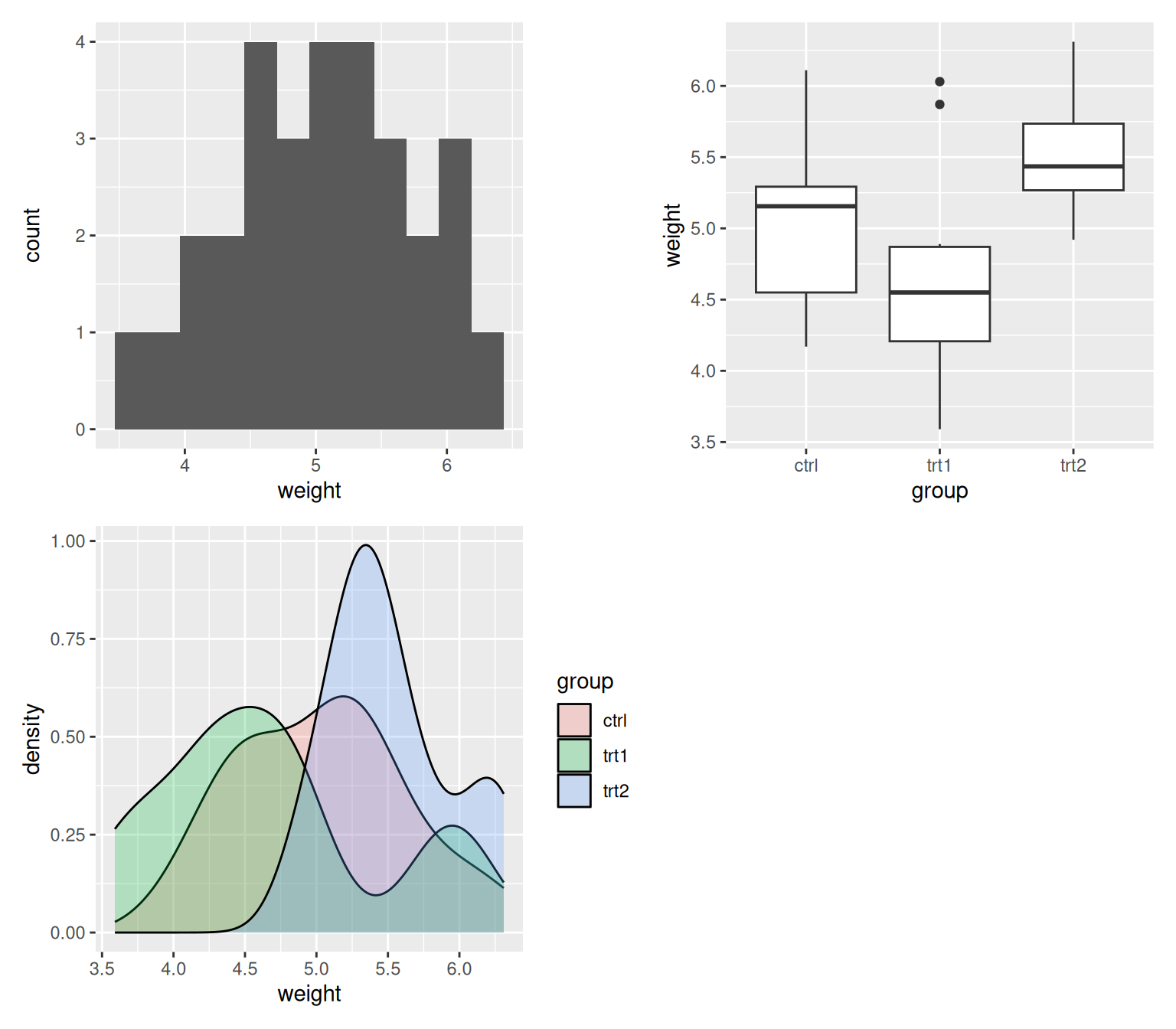## 14.8 Combining Several Plots into the Same Graphic

### 14.8.1 Problem

You want to combine several plots into one graphic output.

### 14.8.2 Solution

The patchwork package can be used to combine several ggplot2 plots into one graphic. (Figure 14.6).

``````library(patchwork)

plot1 <- ggplot(PlantGrowth, aes(x = weight)) +
geom_histogram(bins = 12)

plot2 <- ggplot(PlantGrowth, aes(x = group, y = weight, group = group)) +
geom_boxplot()

plot1 + plot2``````Figure 14.6: Combining two plots together using `patchwork()`

### 14.8.3 Discussion

Patchwork also allows you to determine how you want to lay out the ggplots in relation to each other, by adding a `plot_layout()` call. You can use this call to determine the number of columns you want the pplots to be arranged in (Figure 14.7), and the size of the plots (Figure 14.8):

``````plot3 <- ggplot(PlantGrowth, aes(x = weight, fill = group)) +
geom_density(alpha = 0.25)

plot1 + plot2 + plot3 +
plot_layout(ncol = 2)``````Figure 14.7: Using `plot_layout()` to specify that the plots should be arranged in 2 columns

``````plot1 + plot2 +
plot_layout(ncol = 1, heights = c(1, 4))``````Figure 14.8: Using `plot_layout()` to specify the heights of each plot

In the future, patchwork may be available on CRAN, in which case it can be installed using the usual `install.packages()`.